• 矩阵定义 ...利用MATLAB函数创建矩阵 基本矩阵函数如下： (1) ones()函数：产生全为1的矩阵，ones(n)：产生nn维的全1矩阵，ones(m,n)：产生mn维的全1矩阵； (2) zeros()函数：产生全为0的矩阵； (3) ra
矩阵定义
直接输入法
A=[1 2 3;4 5 6;7 8 9]

矩阵用方括号 “[ ]” 括起
矩阵同一行中的元素之间用 空格 或 逗号 分隔
矩阵行与行之间用 分号  分开
直接输入法中，分号可以用 回车 代替
A=[1 2 3
4 5 6
7 8 9;1 2 3]

由向量生成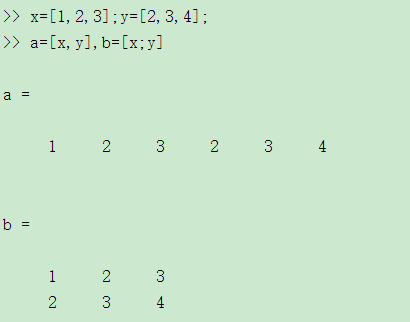通过编写m文件生成
利用MATLAB函数创建矩阵
基本矩阵函数如下：
(1) ones()函数：产生全为1的矩阵，ones(n)：产生nn维的全1矩阵，ones(m,n)：产生mn维的全1矩阵；
(2) zeros()函数：产生全为0的矩阵；
(3) rand()函数：产生在（0，1）区间均匀分布的随机阵；
(4) eye()函数：产生单位阵；
(5) randn()函数：产生均值为0，方差为1的标准正态分布随机矩阵。
(6)tril()下三角矩阵
(7)triu()上三角矩阵
(8)diag（X）：若 X 是矩阵，则 diag(X) 为 X 的主对角线向量
若 X 是向量，diag(X) 产生以 X 为主对角线的对角矩阵
diag(X,k)是提取第k条对角线的元素
使用格式
A=zeros(N) 产生NN的全零矩阵
A=zeros(M,N) 产生MN的全零矩阵
A=zeros(M,N,P,…)产生MNP*…的全零矩阵
A=zeros(siza(b)) 产生和矩阵B维数相同的全零矩阵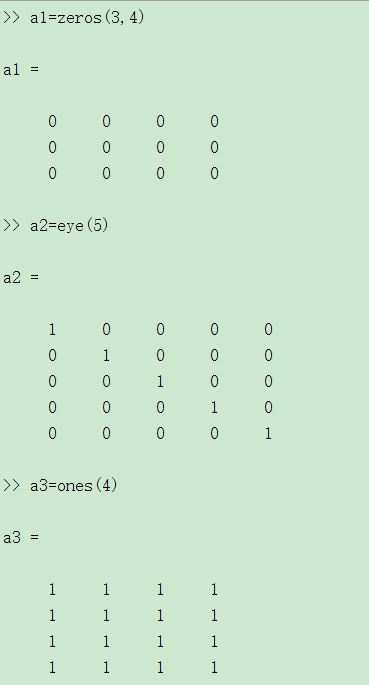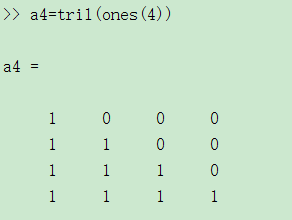生成五阶的随机矩阵，元素在10到90之间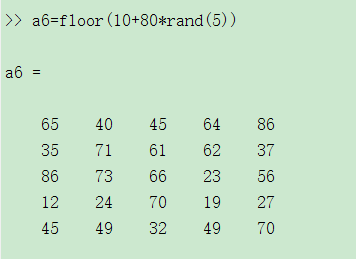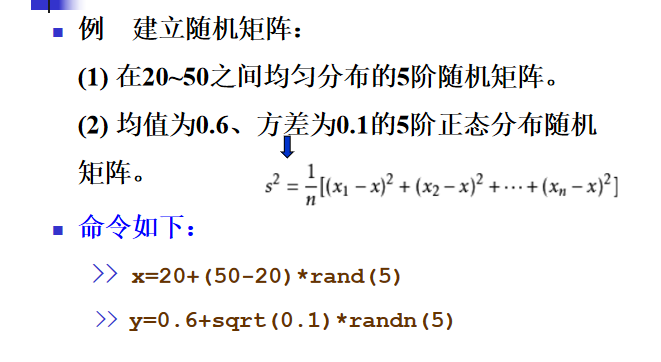矩阵元素赋值
矩阵元素可以是任何形式的表达
x=[-1.3,sqrt(3),(1+2+3)*4/5]

矩阵元素的单独赋值
x(5)=abs(x(1))

Matlab自动将向量 x 的长度扩展到 5，
并将未赋值部分置零。
大矩阵可以把小矩阵作为其元素
A=[A;11 12 13]

矩阵元素的引用
单个元素的引用
x(i)向量x中的第i个元素
A(i,j)矩阵A中的第i行，第j列元素
多个元素的引用：冒号的特殊用法
产生一个由等差序列组成的向量； a 是首项，b 是公差，c 确定最后一项；若 b=1，则 b 可以省略。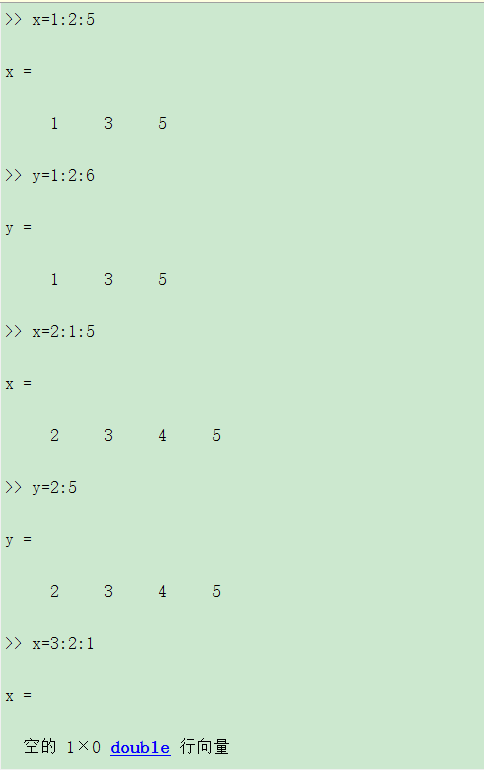A(i:j, m:n) 表示由矩阵 A 的第 i 到第 j 行和第 m 到第 n
列交叉线上的元素组成的子矩阵。
可利用冒号提取矩阵 的整行或整列。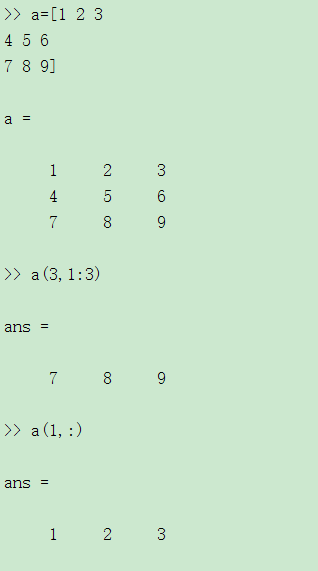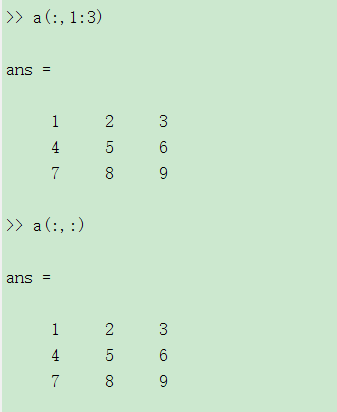用于专门学科的特殊矩阵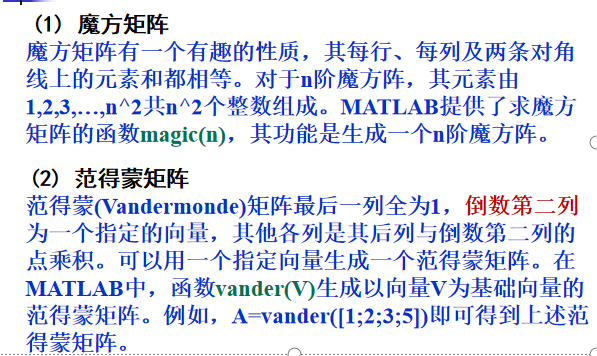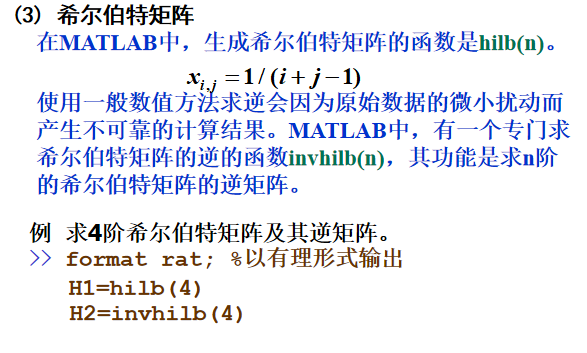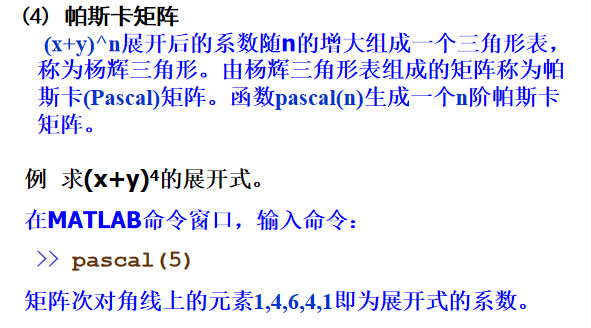矩阵操作
提取矩阵的部分元素： 冒号运算符

A( : )
A的所有元素

A(: , : )
二维矩阵A的所有元素

A(:,k)
A的第K列

A（k,:）
A的第k行

A（k:m）
A的第k个元素到第m个元素

A(:,k:m)
A的第 k 到第 m 列组成的子矩阵

矩阵的旋转

fliplr(A)
左右翻转

flipud(A)
上下翻转

rot90(A)
逆时针旋转90度

rot90(A,k)
逆时针旋转k*90度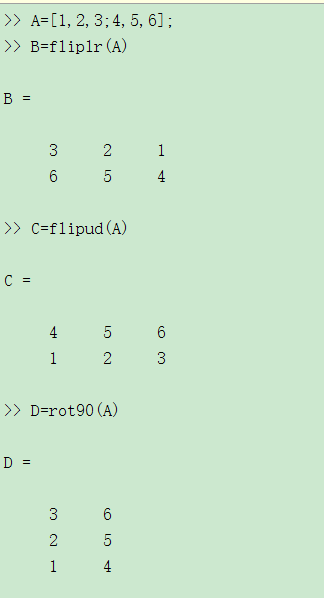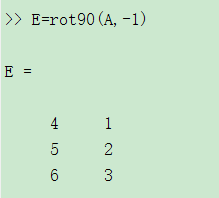矩阵转置与共轭转置
把矩阵A的行和列互相交换所产生的矩阵称为A的转置矩阵，这一过程称为矩阵的转置。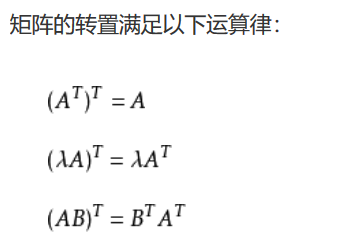矩阵 A 的共轭转置A * 定义为:将矩阵A 的行与列对换，且在将行与列对换时还要将每个元素换成该元素的共轭。

’
共轭转置

.’
转置，矩阵元素不能取共轭

点与单引号之间不能有空格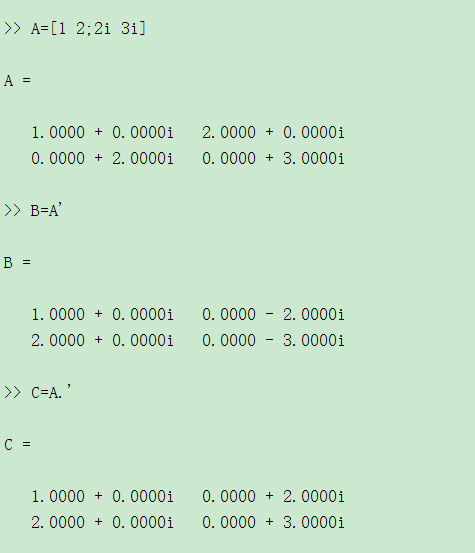矩阵的逆和伪逆
对于一个方阵A,如果存在一个与之同阶方针B，使得AB=BA=E,此时矩阵B为A的逆矩阵，A也是B的逆矩阵，使用函数inv(A)
矩阵的伪逆如果矩阵A不是一个方阵，或者A是一个非满秩的方阵时，矩阵A没有逆矩阵，但可以找到一个与A的转置矩阵A’同型的矩阵B，使得：ABA=A，BAB=B 此时称矩阵B为矩阵A的伪逆，也称为广义逆矩阵。在MATLAB中，求一个矩阵伪逆的函数是pinv(A)。
改变矩阵的形状
reshape(A,m,n)

将矩阵元素按 列方向 进行重组,重组后得到的新矩阵的元素个数必须与原矩阵元素个数相等！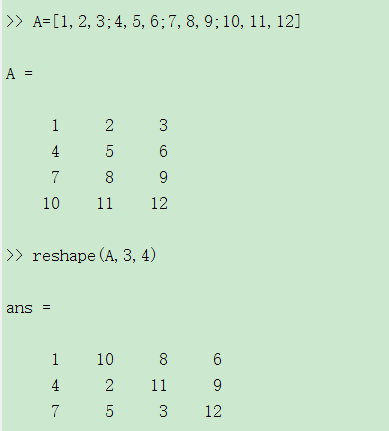查看矩阵的大小

size(A)
列出矩阵的行数和列数

size(A，1）
返回矩阵的行数

size（A,2）
返回矩阵的列数

length(A)
等价于max(size(A))

矩阵的行列式
把一个方阵看作一个行列式，并对其按行列式的规则求值，这个值就称为矩阵所对应的行列式的值。在MATLAB中，求方阵A所对应的行列式的值的函数是det(A)。
矩阵的秩与迹
矩阵的秩 矩阵线性无关的行数与列数称为矩阵的秩。在MATLAB中，求矩阵秩的函数是rank(A)。
矩阵的迹矩阵的迹等于矩阵的对角线元素之和，也等于矩阵的特征值之和。在MATLAB中，求矩阵的迹的函数是trace(A)。
向量和矩阵的范数
矩阵或向量的范数用来度量矩阵或向量在某种意义下的长度。范数有多种方法定义，其定义不同，范数值也就不同。
(1) 向量的3种常用范数及其计算函数 在MATLAB中，求向量范数的函数为：
a、norm(V)或norm(V,2)：计算向量V的2-范数；
b、norm(V,1)：计算向量V的1-范数；
c、norm(V,inf)：计算向量V的∞-范数。
(2) 矩阵的范数及其计算函数 MATLAB提供了求3种矩阵范数的函数，其函数调用格式与求向量的范数的函数完全相同。
(3) 矩阵的条件数 在MATLAB中，计算矩阵A的3种条件数的函数是：
a、cond(A,1) 计算A的1-范数下的条件数；
b、cond(A)或cond(A,2) 计算A的2-范数数下的条件数；
c、cond(A,inf) 计算A的 ∞-范数下的条件数。
矩阵的特征值与特征
向量在MATLAB中，计算矩阵A的特征值和特征向量的函数是eig(A)，常用的调用格式有3种：
(1) E=eig(A)：求矩阵A的全部特征值，构成向量E。
(2) [V,D]=eig(A)：求矩阵A的全部特征值，构成对角阵D，并求A的特征向量构成V的列向量。
(3) [V,D]=eig(A,’nobalance’)：与第2种格式类似，但第2种格式中先对A作相似变换后求矩阵A的特征值和特征向量，而格式3直接求矩阵A的特征值和特征向量。
矩阵的基本运算
矩阵的加减
对应分量进行运算，要求参与加减运算的矩阵具有 相同的维数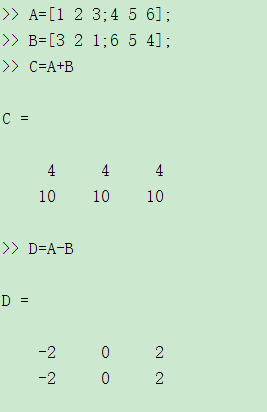矩阵的普通乘法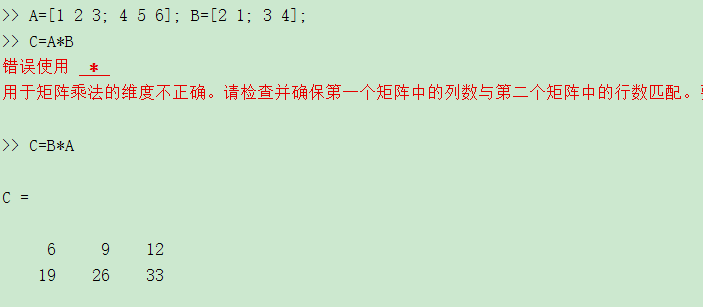矩阵的除法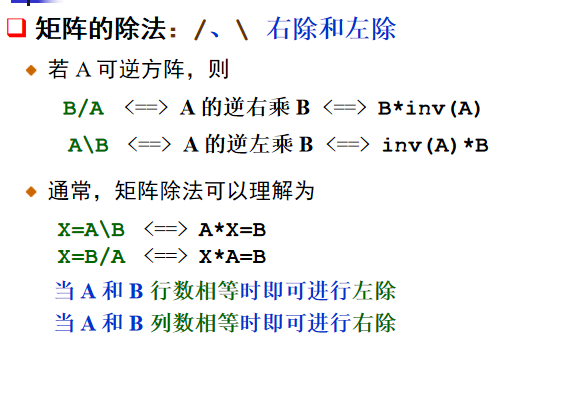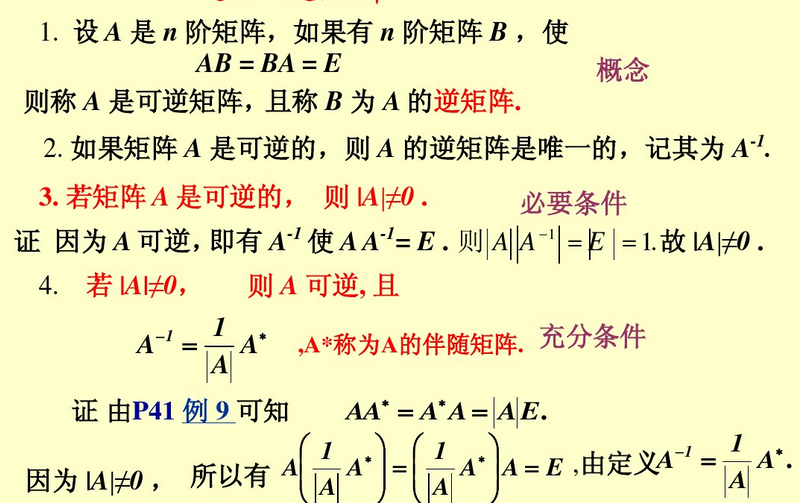矩阵的乘法
A 是方阵，p 是正整数
A^p  表示 A 的 p 次幂，即 p 个 A 相乘。
若 A 是方阵，p 不是正整数
A^p 的计算涉及到 A 的特征值分解，即若
A = VDV-1
则    Ap=V*(D.p)/V
矩阵的 Kronecker 乘积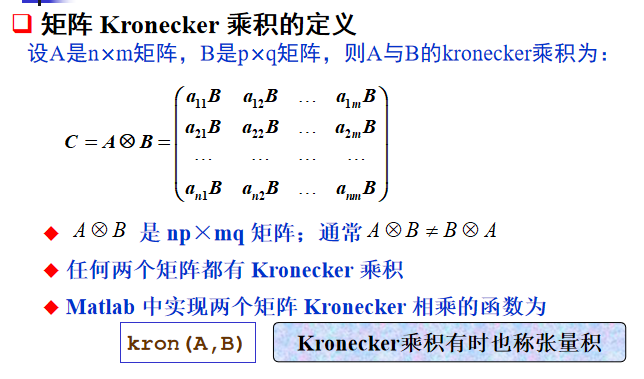矩阵的数组运算
数组运算：对应元素进行运算
数组运算包括：点乘、点除、点幂
相应的数组运算符为： “.* ” ， “./ ” ， “.\ ” 和“ .^ ”
点与算术运算符之间不能有空格！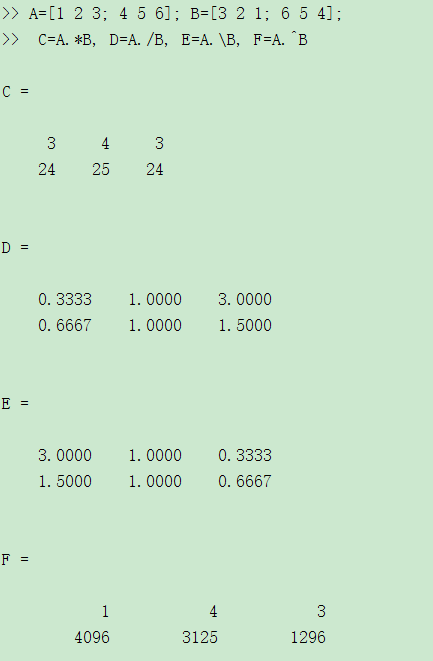矩阵中所有元素
矩阵所有元素求和

sum(A)
对矩阵每一列的元素分别求和

sum(A,2)
对矩阵每一行的元素分别求和

sum(A(😃)
将矩阵的所有元素相加求和

求期望

mean(A)
对矩阵每一列的元素分别求期望

mean(A,2)
对矩阵每一行的元素分别求期望

mean(A(😃)
将矩阵的所有元素相加求期望

求均方差
std2（A）:求矩阵所有元素的均方差
矩阵与数的运算
加减：矩阵的每个元素都与数作加减运算
数乘：矩阵的每个元素都与数作乘法运算
矩阵除以一个数：每个元素都除以这个数
点幂：
底为矩阵，指数为标量
底为标量，指数为矩阵
稀疏矩阵
MATLAB的矩阵有两种存储方式：完全存储方式和稀疏存储方式。
完全存储方式
完全存储方式是将矩阵的全部元素按列存储。以前讲到的矩阵的存储方式都是按这个方式存储的，此存储方式对稀疏矩阵也适用。
稀疏存储方式
稀疏存储方式仅存储矩阵所有的非零元素的值及其位置，即行号和列号。在MATLAB中，稀疏存储方式也是按列存储的。
注意，在讲稀疏矩阵时，有两个不同的概念，一是指矩阵的0元素较多，该矩阵是一个具有稀疏特征的矩阵，二是指采用稀疏方式存储的矩阵。
sparse(S)
将矩阵S转化为稀疏存储方式。当矩阵S已经是稀疏存储方式时，则函数调用相当于ans=S。
例如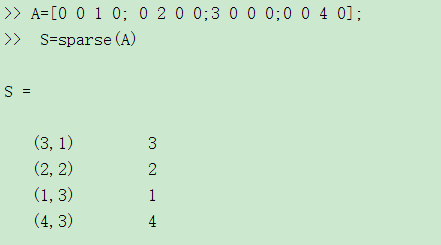sparse函数还有其他一些调用格式：sparse(m,n)：生成一个m×n的所有元素都是0的稀疏矩阵。
sparse(u,v,s,m,n)：u,v,s是3个等长的向量
s是要建立的稀疏矩阵的非0元素
u(i)、v(i)分别是s(i)的行和列标
m,n分别是矩阵的行数和列数。当m,n未被指定时，该函数建立一个max(u)行、max(v)列并以s为稀疏元素的稀疏矩阵
还有一些和稀疏矩阵操作有关的函数。例如[u,v,s]=find(A)：返回矩阵A中非0元素及下标。产生的u,v,s可作为sparse(u,v,s)的参数。full(A)：返回和稀疏存储矩阵A对应的完全存储方式矩阵。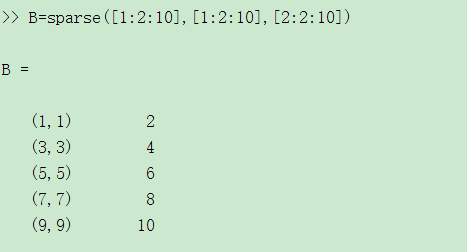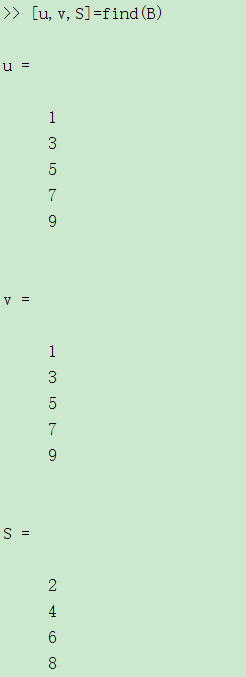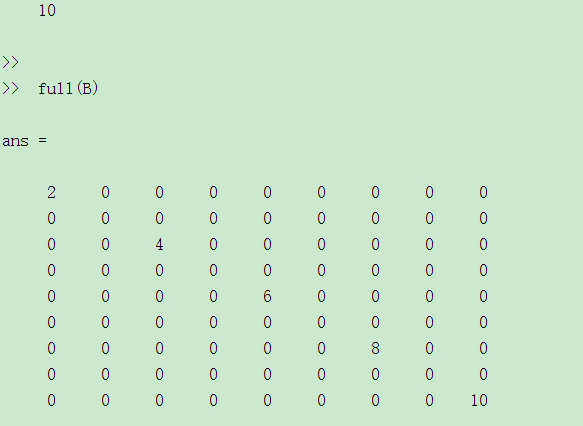特殊稀疏矩阵
单位矩阵只有对角线元素为1，其他元素都是0，是一种具有稀疏特征的矩阵。
函数eye产生一个完全存储方式的单位矩阵。
函数speye(m,n)返回一个m×n的稀疏存储方式的单位矩阵。若m=n可简写为speye(n)。
spones(S):把矩阵S的非零元素值改为1。
sprand：产生非零元素为均匀分布的随机数的稀疏矩阵 。
稀疏存储矩阵只是矩阵的存储方式不同，它的运算规则与普通矩阵是一样的。所以，在运算过程中，稀疏存储矩阵可以直接参与运算。当参与运算的对象不全是稀疏存储矩阵时，所得结果一般是完全存储形式。


展开全文• 伪逆矩阵函数pinv伪逆矩阵MATLAB定义.ppt(2)正交(QR)分解函数矩阵A分解为一个正交矩阵与另一个矩阵的乘积称为矩阵A的正交分解。 格式一：[Q, R]=qr(A) 功能：产生与A同维的上三角矩阵R和一个实正交矩阵或复归...
伪逆矩阵函数pinv伪逆矩阵的MATLAB定义.ppt(2)正交(QR)分解函数  将矩阵A分解为一个正交矩阵与另一个矩阵的乘积称为矩阵A的正交分解。 格式一：[Q, R]=qr(A) 功能：产生与A同维的上三角矩阵R和一个实正交矩阵或复归一化矩阵Q，满足：A=Q*R，Q’*Q=I。 格式二：[Q,R,E]=qr(A) 功能：产生一个置换矩阵E，一个上三角矩阵R(其对角线元素降序排列)和一个归一化矩阵Q，满足A*E=Q*R； (4) 矩阵整体反时针旋转函数rot90( ) 格式一：X=rot90(A) 功能：将矩阵按反时针旋转90o。 格式二：X=rot90(A, k) 功能：将矩阵按反时针旋转k*90o，其中k应为整数。  (5) 对角矩阵和矩阵的对角化函数diag( ) 格式一：X=diag(A,k) 功能：当A为n元向量时，可得n+abs(k)阶的方阵X，其A的元素处于第k条对角线上；k=0表示主对角线，k>0表示在主对角线之上，k<0表示在主对角线之下。当A为矩阵时，X=diag(A,k)得到列向量X，它取自于X的第k个对角线上的元素。 格式二：X=diag(A) 功能：当A为n元向量时，等同于k=0时的X=diag(A,k)，即产生A的元素处于主对角线的对角方阵。当A为矩阵时，X=diag(A)相当于k=0。  (6) 取矩阵的左下三角部分函数tril( ) 格式一：X=tril(A,k) 功能：得到矩阵A的第k条对角线及其以下的元素；当k=0时表示主对角线，k>0表示主对角线之上，k<0表示主对角线以下。 格式二：X=tril(A) 功能：得到矩阵A的下三角阵。 (7) 取矩阵的右上三角部分函数triu( ) 格式一：X=triu(A,k) 功能：得到矩阵A的第k条对角线及其以上的元素；当k=0时表示主对角线，k>0表示主对角线之上，k<0表示主对角线以下。 格式二：X=triu(A) 功能：得到矩阵A的右上三角阵。 (8) 利用“：”将矩阵元素按列取出排成一列 方法：X=A(:)’ (3) 梯形面积法的积分函数trapz( ) 格式一：T=trapz(Y) 功能：以单位间隔，采用计算若干梯形面积的和来计算某函数的近似积分。如果Y为向量，计算Y的积分；如果Y是矩阵，得一个每列积分的行向量；如果Y为多维数组，则沿第一个非单元素维计算。 格式二：T=trapz(X,Y) 功能：用梯形积分法，依据X计算Y的积分。如果X为矢量，则Y必须是同大小的矢量；如果X是一列向量，并且数组Y第一非单元素维长度为length(X)，则在该维中计算。 (4) 双重积分函数dblquad MATLAB提供了一个求双重积分的函数dblquad，其基本调用格式为： 格式：Q=dblquad(fun,xmin,xmax,ymin,ymax,tol) 功能：按指定精度tol，对指定函数 f(x, y)在[xmin, xmax]范围和[ymin, ymax]范围进行双重积分。精度tol缺省时默认精度为1e-6。     * 第 3 章    MATLAB在高等数学中的应用  第3章  MATLAB在高等数学中的应用  3.1   矩阵分析 3.2   多项式运算  3.3   数据的分析与统计  3.4   函数分析与数值积分   3.1 矩阵分析  1．矢量范数和矩阵范数         矩阵范数是对矩阵的一种测度。矢量的p范数和矩阵A的p范数分别定为：          当p＝2时为常用的欧拉范数，一般p还可取l和∞。这在MATLAB中可利用norm函数实现，p缺省时为p=2。 格式：n＝norm(A) 功能：计算矩阵A的最大奇异值，相当于n=max(svd(A))。 格式：n＝norm(A，p) 功能：norm函数可计算几种不同类型的矩阵范数，根据p的不同可得到不同的范数        2．矩阵求逆及行列式值   ⑴矩阵求逆函数inv及行列式值函数det 逆矩阵的定义：对于任意阶 n×n  方阵A，如果能找到一个同阶的方阵V，使得满足：A*V=I。其中I为n阶的单位矩阵eye(n)。则V就是A的逆矩阵。数学符号表示为：V=A-1。逆矩阵V存在的条件是A的行列式不等于0。 格式：V=inv(A) 功能：返回方阵A的逆矩阵V。 格式：X=det(A) 功能：计算方阵A的行列式值。       ⑵伪逆矩阵函数pinv 伪逆矩阵的MATLAB定义：从数学意义上讲，当矩阵A为非方阵时，其矩阵的逆是不存在的。在MATLAB中，为了求线性方程组的需要，把inv(A′*A)*A′的运算定义为伪逆函数pinv，这样对非方阵，利用伪逆函数pinv可以求得矩阵的伪逆，伪逆在一定程度上代表着矩阵的逆。 格式：C=pinv(A) 功能：计算非方阵A的伪逆矩阵。 3．线性代数方程求解  写成矩阵形式可表示为：AX＝B  或
展开全文• 首先普及一下LTI各类对象之间可以进行相互转换，下面列出这些转换函数的调用格式。1) sys=tf(sys) %将sys对象转换为TF模型2) sys=zpk(sys) %转换为ZPK模型3)sys=ss(sys) %转换为SS模型4)sys=frd(sys,frequency) %...
首先普及一下LTI各类对象之间可以进行相互转换，下面列出这些转换函数的调用格式。1) sys=tf(sys) %将sys对象转换为TF模型2) sys=zpk(sys) %转换为ZPK模型3)sys=ss(sys) %转换为SS模型4)sys=frd(sys,frequency) %转换为FRD模型步入正题。第一种方法设系统的矩阵分别为A=[2,0,0;0,4,1;0,0,4];B=[1,0,1]';C=[1,1,0];D=0求取该系统的TF模型，如下图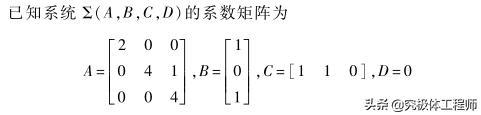例1实现程序如下：A=[2,0,0;0,4,1;0,0,4];B=[1,0,1]';C=[1,1,0];D=0;[num,den]=ss2tf(A,B,C,D);G=tf(num,den)结果如下图：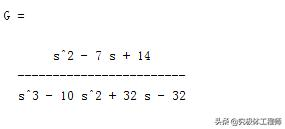结果第二种方法依旧借用例1，实现的程序如下：A=[2,0,0;0,4,1;0,0,4];B=[1,0,1]';C=[1,1,0];D=0;gss=ss(A,B,C,D);gtf=tf(gss);结果同方法一结果一样。第三种方法最后一种方法利用自控原理线性系统的传递函数公式求取，公式为：C*inv(SI-A)*B此方法可以用来验证上述两种方法求取的传递函数正确性，对比此方法与上述两种方法有什么区别。实现程序如下：syms s GG gg;A=[2,0,0;0,4,1;0,0,4];B=[1,0,1]';C=[1,1,0];D=0;G=C*inv(s*eye(3)-A)*Bgg=simplify(GG);结果如下图;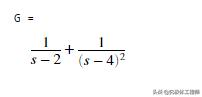结果经过通分之后，可以发现此结果与前两种方法一样。经过上面的分析，上面三种方法根据各自喜好选择，各有各的优点。另外再补充几个LTI各对象之间的转换调用格式[z,p,k]=tf2zp(num,den) %将TF对象的属性转换为ZPK对象的属性[A,B,C,D]=tf2ss(num,den) %将TF对象的属性转换为SS对象属性[num,den]=zp2tf(z,p,k) %将ZPK对象的属性转换为TF对象的属性[A,B,C,D]=zp2ss(z,p,k) %将ZPK对象的属性转换为SS对象的属性[z,p,k]=ss2zp(A,B,C,D,iu) %将SS对象的属性转换为ZPK对象的属性
展开全文• Matlab提供了强大的函数库供用户调用，但也支持用户自定义函数。本文使用了范德堡大学教授Akos Ledeczi授课中的样例来一步步说明怎样在Matlab中自定义函数。 首先，在command window中输入edit命令。開始编辑...


Matlab提供了强大的函数库供用户调用，但也支持用户自定义函数。本文使用了范德堡大学教授Akos Ledeczi授课中的样例来一步步说明怎样在Matlab中自定义函数。

首先，在command window中输入edit命令。開始编辑函数。本函数实现的是随机生成一个3行4列的矩阵。该矩阵的元素取值范围在0-1之间。

function myRand
a = rand(3,4)
end当中，function和end是keyword，function表示该文件是一个函数，end表示函数体的结束。在command window中运行myRand命令，得到的结果例如以下：

>> myRand
a =
0.5688    0.3371    0.3112    0.6020
0.4694    0.1622    0.5285    0.2630
0.0119    0.7943    0.1656    0.6541
然后，为函数添加參数。本函数实现的是随机生成一个3行4列的矩阵，该矩阵的元素取值范围在low-high之间。

function a = myRand(low, high)
a = low+rand(3,4)*(high - low);
end当中。low和high是函数体的两个输入參数。分别代表了矩阵元素取值范围的上限和下限；a是函数的范围值。在command window中运行myRand命令。得到的结果例如以下：>> myRand(1,6)
ans =
4.4461    1.4191    1.7619    5.9807
4.7408    2.1449    5.1291    1.3909
3.2527    5.5667    3.6917    3.2134

最后，为函数再添加一个返回參数。该函数将随机生成的矩阵中的每个元素相加。返回其总和。

function [a, s] = myRand(low, high)
a = low + rand(3,4)*(high-low);
v = a(:);
s = sum(v);
end当中。s是新添加的返回參数。在command window中运行myRand命令，得到的结果例如以下：

>> [x ss] = myRand(2,4)
x =
3.1504    2.7063    2.0860    3.4634
2.1196    3.6424    2.3380    3.2955
2.4696    2.0308    3.2982    2.9018
ss =
33.5021
到此为止。在Matlab中简单自己定义函数的方法已经介绍完成。Matlab还支持常见的for-loop和if-else等循环和推断结构。參见兴许博客。

转载于:https://www.cnblogs.com/lcchuguo/p/5221815.html
展开全文• 函数文件的基本结构 function 输出形参表=函数名（输入形参表） 注释说明部分 函数体语句 注意：当多个形参时，形参之间用逗号分隔，组成形参表。当输出形参多于一个时，应该用方...函数文件名与函数名自己定义 ...
• matlab函数大全-matlab函数大全.doc 比较白痴的东西，不过对初学者也许有用，特分享一下。不要喷我哦!函数具体用法可以用help查一下。附件内容与下面一样的。 Aabs 绝对值、模、字符的ASCII码值 acos 反余弦 ...
• 1、定义矩阵、 2、转置矩阵、 3、矩阵放到一列、 4、逆矩阵、 二、随机数函数、 1、rand 随机数函数、 2、randn 随机数函数、 3、randi 随机数函数、 三、生成矩阵、 1、生成 0 矩阵、 2、生成随机矩阵、
• 协方差矩阵 cov(X) 相关系数矩阵 corrcoef(X) 设原矩阵 X（n,p），标准化矩阵 Xstd（n,p），则有： 1.标准化矩阵的协方差矩阵 cov（Xstd） == 原数据矩阵的相关系数矩阵corrcoef（X）； 2.标准化矩阵转置乘本身 ...
• 上期我们简单介绍了矩阵的生成方式，这里详细说明矩阵的基本定义。首先矩阵大小可以用“size”函数查看，其中返回结果中第一个数字代表行数，第二个数字代表列数，第三个数字代表第三维，后面的数字以此类推。当需要...索引超出矩阵维度
• ## MATLAB01:基本的数学运算与矩阵运算

万次阅读 多人点赞 2019-11-12 17:22:52
基本的数学运算与矩阵运算MATLAB基本语法变量变量名保留变量不适合做变量...函数使用MATLAB进行矩阵运算定义矩阵向终端输入矩阵使用冒号运算符创建向量定义特殊矩阵矩阵的索引矩阵的操作操作矩阵的运算符操作矩阵的...
• 定义 定理：若n*n阶矩阵A是对称正定的，则存在一个对角元为正数的下三角矩阵L，使得A = L’*L成立（其中L‘表示L的共轭转置）。 用法 R=chol（A） chol（A）只使用A的对角线和上三角形。下三角假设为上三角的（复...
• b = 2; c = 3.5; x1 = fzero(@(x) cubicpoly(x,b,c),0) x2 = fzero(@cubicpoly,0,[],b,c) function y = cubicpoly(x,b,c) ...匿名矩阵的传递参数的思路是再次定义一个函数，将参数传入。 第一种方法比较传
• matlab支持多输入多输出，函数的返回值可以为一个向量，矩阵，或者多个元素。为了让函数可以调用，需要对函数进行命名。 function [输出变量] = 函数名称(输入变量） 即包含： 输入参数 输出参数 函数名 注释 ...
• ## Matlab定义子函数

千次阅读 2016-01-24 08:38:00
上篇博客介绍了在Matlab中自己定义简单函数的方法，本篇博客将介绍定义子函数的方法。本文承接上篇博客的样例，即随机生成一个3行4列的矩阵矩阵中的元素设定上下限为（low，high）。并返回矩阵全部元素的和。.m...
• 一、协方差矩阵定义及其计算公式 　协方差矩阵在机器学习中经常用到，查看wiki：http://zh.wikipedia.org/wiki/%E5%8D%8F%E6%96%B9%E5%B7%AE%E7%9F%A9%E9%98%B5 可知协方差矩阵的具体计算公式如下： 在统计学与...
• MATLAB中创建矩阵有以下规则： a、矩阵元素必须在”[ ]”内； b、矩阵的同行元素之间用空格或逗号隔开； c、矩阵的行与行之间用”;”（或回车符）隔开； d、矩阵的元素可以是数值、变量、表达式或函数
• 新建一个.m文件，复制以下代码即可定义函数PlotConfusion，直接按要求调用即可： function F1=PlotConfusion(confusion_matrix) %输入混淆矩阵，绘制混淆矩阵图 %列的方向是真实值，行的方向是预测值 %或者说是x轴为...机器学习
• ## matlab函数 kron

千次阅读 2016-06-29 10:38:36
matlab函数 kron 格式 C=kron(A,B) %A为m×n矩阵，B为p×q矩阵，则C为mp×nq矩阵。 kron即为Kronecker积，所谓Kronecker积是一种矩阵运算，其定义可以简单描述成： X与Y的Kronecker积的结果是一个矩阵： X11*
• QT / C++ / Eigen 自己实现的MATLAB中的std()函数矩阵按照列求标准差 //定义一个按列求标准差的函数，其中A为被求列标准差的矩阵，OutputMatrix为求出的列标准差 void Std(MatrixXd &A, MatrixXd &...qt c++
• 目录前言数组的定义与生成下一篇预告前言首先总结一下,前面几篇已经介绍了Matlab在高等数学上、下册的基本应用，包括(点击红色字体可以查看链接)：“符号表达式，函数求极限，泰勒展开，函数求导，一元函数求积分”...
• MATLAB中创建矩阵有以下规则： a、矩阵元素必须在”[ ]”内； b、矩阵的同行元素之间用空格（或”,”）隔开； c、矩阵的行与行之间用”;”（或回车符）隔开； d、矩阵的元素可以是数值、变量、表达式或函数； ...
• 坑爹的最优化算法要求学习Matlab，赶紧自学 一、矩阵的表示 在MATLAB中创建矩阵有...d、矩阵的元素可以是数值、变量、表达式或函数； e、矩阵的尺寸不必预先定义。 二，矩阵的创建： 1、直接输入法
• 稀疏矩阵定义：即其中只有很少非零元素的矩阵，这样的矩阵就成为稀疏矩阵，这种特性提供了矩阵存储空间和...稀疏矩阵的转换：给出一个矩阵A，我们可以使用MATLAB函数sparse把它转换成稀疏矩阵，该函数语法为：　S＝spa
• 不仅有函数的基本形式，还有对应的matlab函数，用起来很方便。 Eigen 矩阵定义 #include <Eigen/Dense> Matrix<double, 3, 3> A; // Fixed rows and cols. Same as Matrix3d. Matrix...
• MATLAB中创建矩阵有以下规则： a、矩阵元素必须在”[ ]”内； b、矩阵的同行元素之间用空格（或”,”）隔开； c、矩阵的行与行之间用”;”（或回车符）隔开； d、矩阵的元素可以是数值、变量、表达式...
• d、矩阵的元素可以是数值、变量、表达式或函数； e、矩阵的尺寸不必预先定义。 二，矩阵的创建： 1、直接输入法 最简单的建立矩阵的方法是从键盘直接输入矩阵的元素，输入的方法按照上面的规则......

# matlab函数定义矩阵matlab 订阅